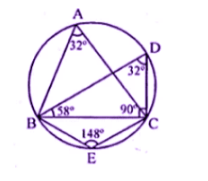Guru

# Question 3. (a) In the figure, (i) given below, if ∠DBC = 58° and BD is a diameter of the circle, calculate: (i) ∠BDC (ii) ∠BEC (iii) ∠BAC

• 0

This is an important and exam oriented question from Chapter name- circles
Topic – Angle properties of circles
Chapter number- 15

In this question we have been given that ∠DBC = 58° and BD is a diameter of the circled

And we hve to , calculate:

(i) ∠BDC (ii) ∠BEC (iii) ∠BAC
ICSE Avichal publication
Understanding ICSE Mathematics
Question no 3(a)

Share

1. Solution:

(a) ∠DBC = 58°

BD is diameter

∠DCB = 90° (Angle in semi-circle)

(i) In ∆BDC

∠BDC + ∠DCB + ∠CBD = 180°

∠BDC = 180°- 90° – 58° = 32°(ii) BEC = 180o – 32= 148o

(opposite angles of cyclic quadrilateral)

(iii) ∠BAC = ∠BDC = 32o

(Angles in same segment

• 0